## Airy Functions

Watson's (1966, pp. 188-190) definition of an Airy function is the solution to the Airy Differential Equation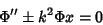(1)

which is Finite at the Origin, where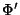denotes the Derivative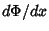,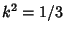, and either Sign is permitted. Call these solutions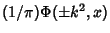, then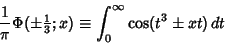(2)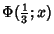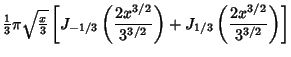(3)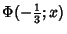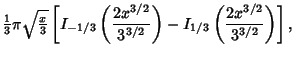(4)

whereis a Bessel Function of the First Kind and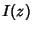is a Modified Bessel Function of the First Kind. Using the identity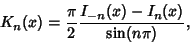(5)

where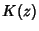is a Modified Bessel Function of the Second Kind, the second case can be re-expressed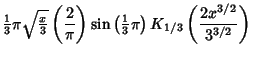(6)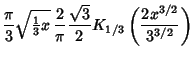(7)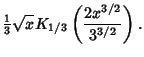(8)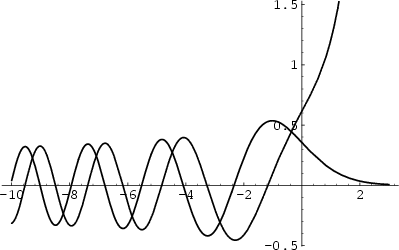A more commonly used definition of Airy functions is given by Abramowitz and Stegun (1972, pp. 446-447) and illustrated above. This definition identifies the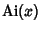and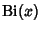functions as the two Linearly Independent solutions to (1) with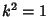and a Minus Sign,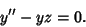(9)

The solutions are then written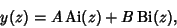(10)

where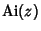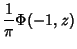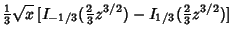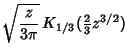(11)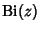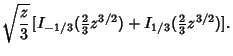(12)

In the above plot,is the solid curve andis dashed. For zero argument,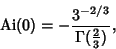(13)

where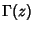is the Gamma Function. This means that Watson's expression becomes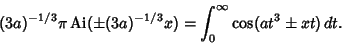(14)

A generalization has been constructed by Hardy.

The Asymptotic Series ofhas a different form in different Quadrants of the Complex Plane, a fact known as the Stokes Phenomenon. Functions related to the Airy functions have been defined as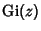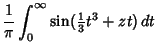(15)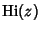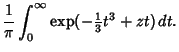(16)

References

Abramowitz, M. and Stegun, C. A. (Eds.). Airy Functions.'' §10.4 in Handbook of Mathematical Functions with Formulas, Graphs, and Mathematical Tables, 9th printing. New York: Dover, pp. 446-452, 1972.

Press, W. H.; Flannery, B. P.; Teukolsky, S. A.; and Vetterling, W. T. Bessel Functions of Fractional Order, Airy Functions, Spherical Bessel Functions.'' §6.7 in Numerical Recipes in FORTRAN: The Art of Scientific Computing, 2nd ed. Cambridge, England: Cambridge University Press, pp. 234-245, 1992.

Spanier, J. and Oldham, K. B. The Airy Functions Ai() and Bi().'' Ch. 56 in An Atlas of Functions. Washington, DC: Hemisphere, pp. 555-562, 1987.

Watson, G. N. A Treatise on the Theory of Bessel Functions, 2nd ed. Cambridge, England: Cambridge University Press, 1966.